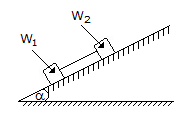# Civil Engineering - UPSC Civil Service Exam Questions

26.

In the figure shows two blocks of equal weights designated as W1 and W2 connected together by a rod and resting on an inclined plane whose angle α can be varied. The coefficients of friction between the blocks and the surface of the plane are μ1 = 0.2 and μ2 = 0.3. The value of angle α at which the slipping will commence is given byA. tan α = 0.1 B. tan α = 0.2 C. tan α = 0.25 D. tan α = 0.8

Explanation:

No answer description available for this question. Let us discuss.

27.

The most suitable type of equipment for compaction of cohesive soils is

 A. Smooth-wheeled rollers B. Vibratory rollers C. Sheep foot rollers D. Tampers

Explanation:

No answer description available for this question. Let us discuss.

28.

Ringelmann's scale is used to

 A. measure CO B. measure SO2 C. grade density of smoke D. grade automobile exhaust gas

Explanation:

No answer description available for this question. Let us discuss.

29.

Consider the following statements :
1. Obtaining reliable properties of soil is very important in geotechnical engineering. Chunk
samples obtained from the field are the best for this purpose.
2. Chunk samples may easily be obtained at all depths below ground level and for all types of soils.
3. Undisturbed soil samples are enclosed in rugged containers. As such, they do not get disturbed easily during transportation and handling.
Which of the statements given above is/are correct ?

 A. 1, 2 and 3 B. 1 only C. 2 and 3 D. 1 and 3

Explanation:

No answer description available for this question. Let us discuss.

30.

In a V-notch, an error of 1% in the measurement of head would constitute in the discharge measurement, an error of

 A. 1.0% B. 1.5% C. 2.0% D. 2.5%